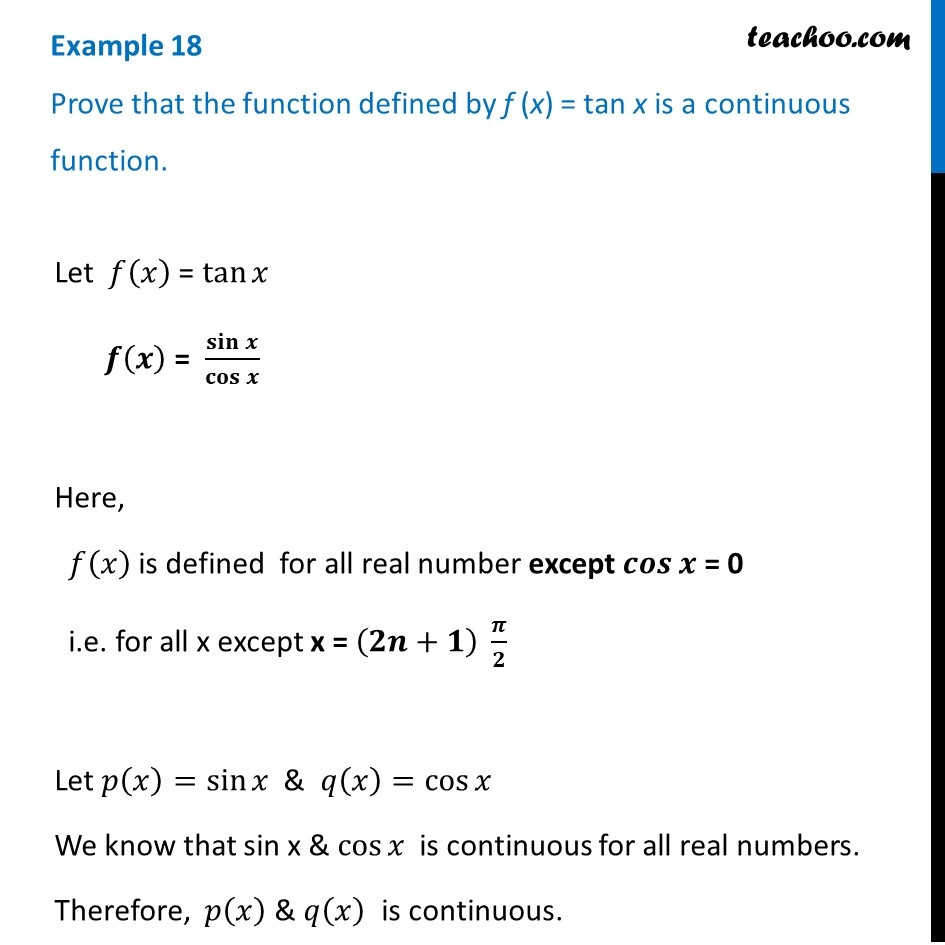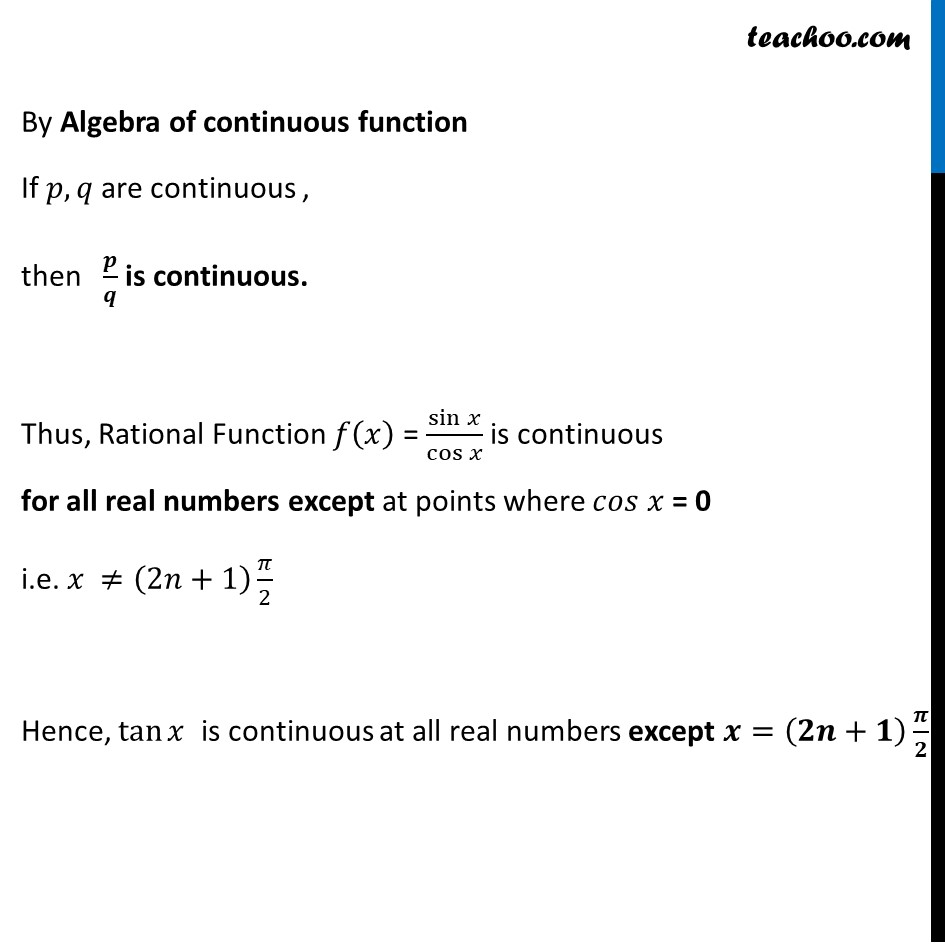1. Chapter 5 Class 12 Continuity and Differentiability (Term 1)
2. Serial order wise
3. Examples

Transcript

Example 18 Prove that the function defined by f (x) = tan x is a continuous function.Let 𝑓(𝑥) = tan⁡𝑥 𝒇(𝒙) = 𝐬𝐢𝐧⁡𝒙/𝐜𝐨𝐬⁡𝒙 Here, 𝑓(𝑥) is defined for all real number except 𝒄𝒐𝒔⁡𝒙 = 0 i.e. for all x except x = (𝟐𝒏+𝟏) 𝝅/𝟐 Let 𝑝(𝑥)=sin⁡𝑥 & 𝑞(𝑥)=cos⁡𝑥 We know that sin x & cos⁡𝑥 is continuous for all real numbers. Therefore, 𝑝(𝑥) & 𝑞(𝑥) is continuous. By Algebra of continuous function If 𝑝, 𝑞 are continuous , then 𝒑/𝒒 is continuous. Thus, Rational Function 𝑓(𝑥) = sin⁡𝑥/cos⁡𝑥 is continuous for all real numbers except at points where 𝑐𝑜𝑠 𝑥 = 0 i.e. 𝑥 ≠(2𝑛+1) 𝜋/2 Hence, tan⁡𝑥 is continuous at all real numbers except 𝒙=(𝟐𝒏+𝟏) 𝝅/𝟐

Examples

Chapter 5 Class 12 Continuity and Differentiability (Term 1)
Serial order wise